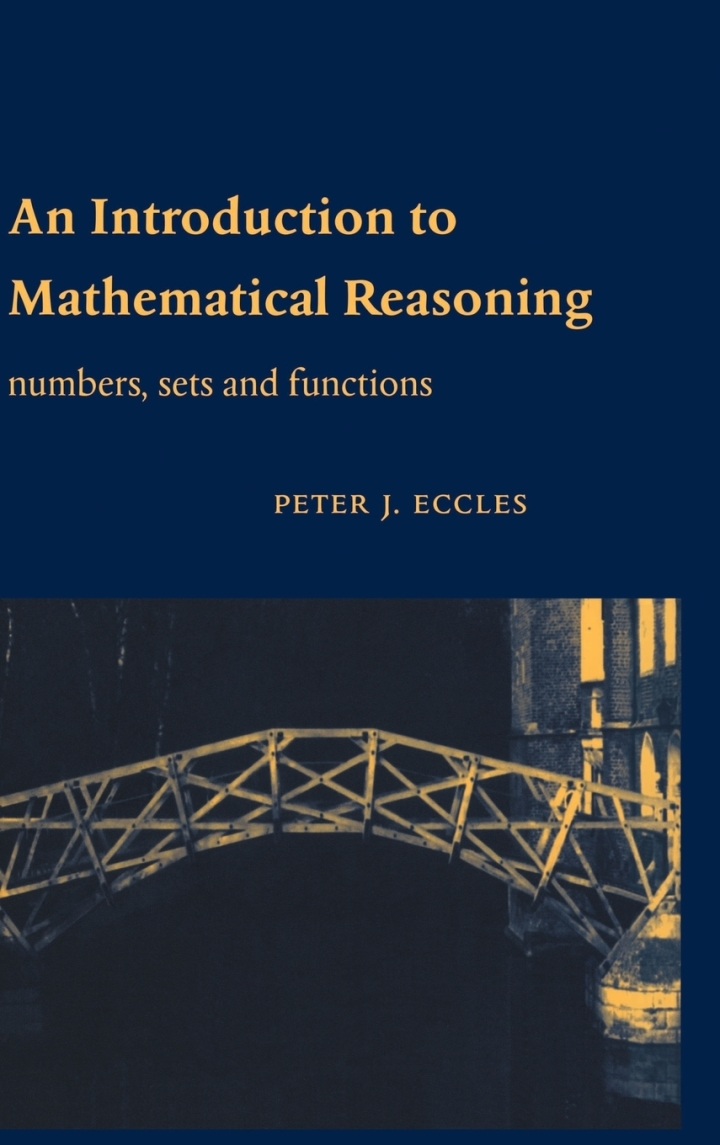# An Introduction to Mathematical Reasoning

## An Introduction to Mathematical Reasoning

By Peter J. Eccles

### Rent or Buy eTextbook

Expires on Apr 22nd, 2022
\$37

Publisher List Price: \$0.00

The purpose of this book is to introduce the basic ideas of mathematical proof to students embarking on university mathematics. The emphasis is on helping the reader in understanding and constructing proofs and writing clear mathematics. This is achieved by exploring set theory, combinatorics and number theory, topics which include many fundamental ideas which are part of the tool kit of any mathematician. This material illustrates how familiar ideas can be formulated rigorously, provides examples demonstrating a wide range of basic methods of proof, and includes some of the classic proofs. The book presents mathematics as a continually developing subject. Material meeting the needs of readers from a wide range of backgrounds is included. Over 250 problems include questions to interest and challenge the most able student as well as plenty of routine exercises to help familiarize the reader with the basic ideas.

Subject: Mathematics & Statistics -> Post-Calculus -> LogicNumbers, Sets and Functions
1st edition
Publisher: Cambridge University Press 12/11/97
Imprint: Cambridge University Press
Language: English

ISBN 10: 1139636316
ISBN 13: 9781139636315
Print ISBN: 9780521592697

Live Chats